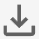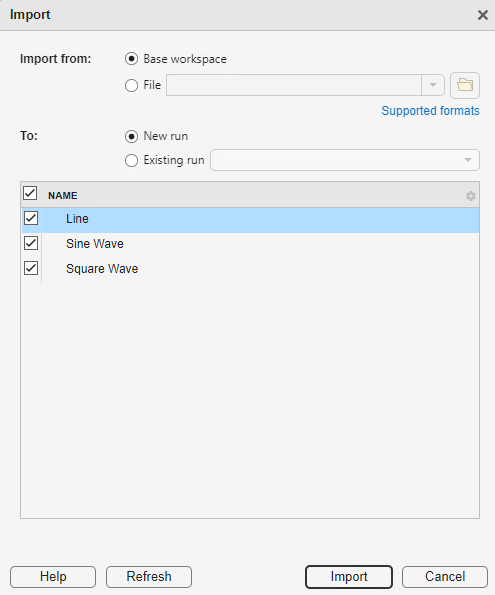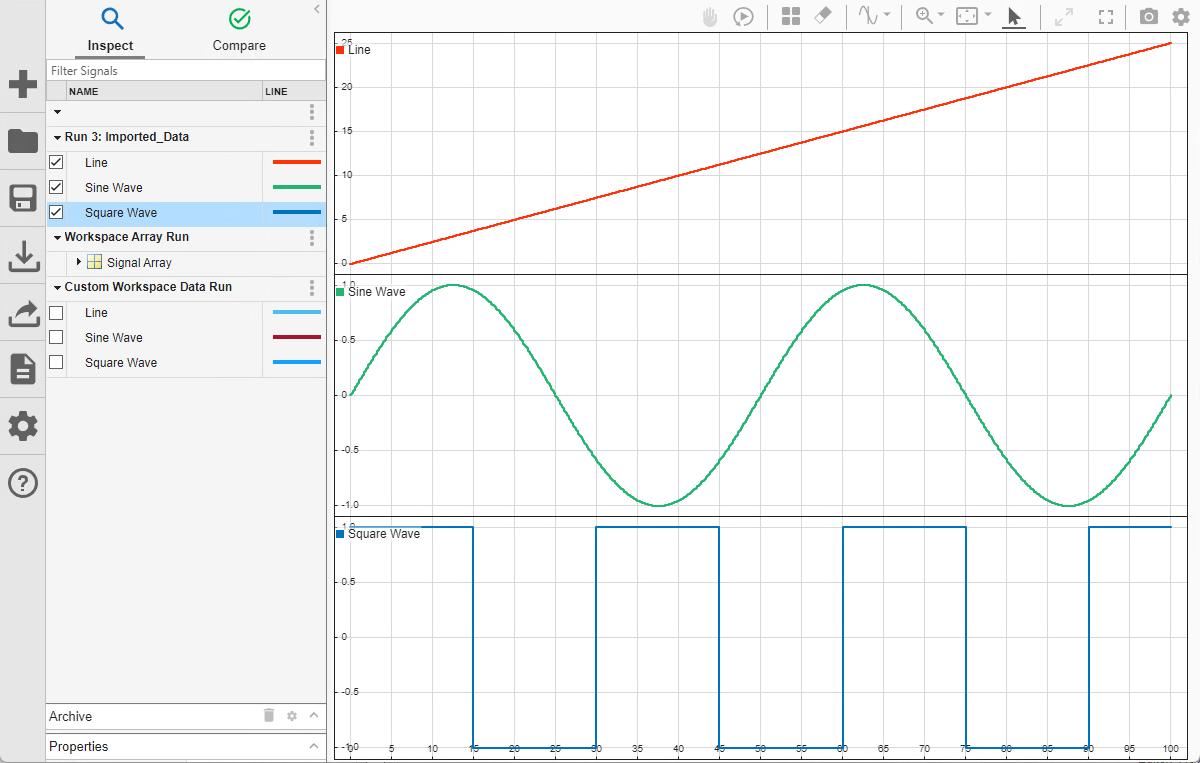# Import Workspace Variables Using a Custom Data Reader

When your workspace data is in a format that built-in readers do not support, you can write a custom data reader to import the data into the Simulation Data Inspector. This example explains the parts of the class definition for a custom workspace reader and shows how to register the reader with the Simulation Data Inspector. Open the `SimpleStructReader.m` file to view the complete class definition.

### Create Workspace Data

First, create workspace data to import into the Simulation Data Inspector using the custom reader. Suppose you store each signal as a structure with fields for the data (`d`), the time values (`t`), and the signal name (`n`).

```time = 0:0.1:100; time = time'; lineData = 1/4*time; sineWave = sin((2*pi)/50*time); squareWave = square((2*pi)/30*time); mySineVar.d = sineWave; mySineVar.t = time; mySineVar.n = "Sine Wave"; myLineVar.d = lineData; myLineVar.t = time; myLineVar.n = "Line"; mySquareVar.d = squareWave; mySquareVar.t = time; mySquareVar.n = "Square Wave";```

### Write the Class Definition for a Custom Reader

Write a class definition that specifies how the custom reader extracts relevant data and metadata from the workspace variables. Save the class definition file in a location on the MATLAB™ path.

The class definition starts by inheriting from the `io.reader` class, followed by property and method definitions. The custom reader in this example defines the property `ChannelIndex`. You can use the reader to import individual structures or an array of structures from the workspace. The `ChannelIndex` property is used when importing an array of structures.

```classdef SimpleStructReader < io.reader properties ChannelIndex end ```

Every custom reader must define the `getName`, `getTimeValues`, and `getDataValues` methods. When you write a custom reader to import data from the workspace, you must also define the `supportsVariable` method. The reader in this example also defines the `getChildren` method to support importing an array of structures.

The `supportsVariable` method checks which variables in the workspace are supported by the reader. In this example, the `supportsVariable` returns `true` when:

1. The structure contains the appropriate fields.

2. The `n` field of the structure contains a string or character array that represents the signal name.

3. The `t` field of the structure is a column vector of double data.

4. The `d` field contains numeric data.

5. The `d` field is the same size as the `t` field, meaning there is a sample value for each time step.

```function supported = supportsVariable(~, val) % Support structure with fields t (time), d (data), and n (name) supported = ... isstruct(val) && ... isfield(val,'t') && ... isfield(val,'d') && ... isfield(val,'n'); if supported for idx = 1:numel(val) varName = val(idx).n; time = val(idx).t; varData = val(idx).d; % Name must be string or character array if ~ischar(varName) && ~isstring(varName) supported = false; % Time must be double column vector elseif ~isa(time,'double') || ~iscolumn(time) supported = false; % Data size must match time size else timeSz = size(time); dataSz = size(varData); if ~isnumeric(varData) || ~isequal(dataSz, timeSz) supported = false; end end end end end ```

The `getChildren` method creates a `SimpleStructReader` object for each structure in an array of structures. When the variable to import is not scalar, the `getChildren` method assigns a value to the `ChannelIndex` property added to the class for the custom reader. The `VariableValue` property for each `SimpleStructReader` object returned by the `getChildren` method is the array of structures. Other methods use the `ChannelIndex` property to extract the appropriate signal name, signal data, and time values from each object.

```function childObj = getChildren(obj) childObj = {}; if ~isscalar(obj.VariableValue) && isempty(obj.ChannelIndex) numChannels = numel(obj.VariableValue); childObj = cell(numChannels,1); for idx = 1:numChannels childObj{idx} = SimpleStructReader; childObj{idx}.VariableName = sprintf('%s(%d)',obj.VariableName,idx); childObj{idx}.VariableValue = obj.VariableValue; childObj{idx}.ChannelIndex = idx; end end end ```

The `getName` method assigns the name stored in the `n` field of the structure to each imported signal. When the imported variable is scalar, the method gets the name from the `VariableValue` property of the `SimpleStructReader` object. When the imported data is an array of structures, the appropriate structure is extracted from the `VariableValue` property using the `ChannelIndex` property. The top-level node of the array is named `Signal Array`.

```function retName = getName(obj) if isscalar(obj.VariableValue) retName = char(obj.VariableValue.n); elseif ~isempty(obj.ChannelIndex) varVal = obj.VariableValue(obj.ChannelIndex); retName = char(varVal.n); else retName = 'Signal Array'; end end ```

The `getTimeVals` and `getDataVals` methods handle scalar and nonscalar structures similar to how the `getName` method does. For a scalar structure, both methods extract the appropriate field from the `VariableValue` property of the `SimpleStructReader` object. For a nonscalar structure, both methods access the appropriate structure in the `VariableValue` property using the `ChannelIndex` property. Finally, for the top-level node of the array, time and data are both returned as empty.

```function timeVals = getTimeValues(obj) if isscalar(obj.VariableValue) timeVals = obj.VariableValue.t; elseif ~isempty(obj.ChannelIndex) varVal = obj.VariableValue(obj.ChannelIndex); timeVals = varVal.t; else timeVals = []; end end function dataVals = getDataValues(obj) if isscalar(obj.VariableValue) dataVals = obj.VariableValue.d; elseif ~isempty(obj.ChannelIndex) varVal = obj.VariableValue(obj.ChannelIndex); dataVals = varVal.d; else dataVals = []; end end ```

After you write the class definition for the custom reader, you must register the reader before you can use it to import data into the Simulation Data Inspector. The Simulation Data Inspector does not store registered readers between MATLAB sessions, so you need to register a custom reader at the start of each new MATLAB session. To register the workspace data reader in this example, use the `registerWorkspaceReader` method.

`registerWorkspaceReader(SimpleStructReader);`

To confirm that the reader is registered, use the `io.reader.getRegisteredWorkspaceReaders` method.

`io.reader.getRegisteredWorkspaceReaders`
```ans = "SimpleStructReader" ```

### Import Workspace Data in a Custom Format

Once you register the custom workspace data reader, you can import workspace variables stored using the custom format into the Simulation Data Inspector using the UI or the `Simulink.sdi.createRun` function.

To import data using the UI, open the Simulation Data Inspector. You can use the `Simulink.sdi.view` function to open the Simulation Data Inspector from the MATLAB Command Window. Then, select Import.The Import dialog shows the data in the base workspace that the Simulation Data Inspector is able to import using built-in and registered custom readers. Because the custom reader is registered, the `Line`, `Sine Wave`, and `Square Wave` signals are available for import, while the `lineData`, `sineWave`, and `squareWave` variables are not. Select the data you want to import and select Import. The data imports into a run called `Imported_Data`.To import data from the workspace programmatically, use the `Simulink.sdi.createRun` function.

`Simulink.sdi.createRun('Custom Workspace Data Run','vars',myLineVar,mySineVar,mySquareVar);`

The custom reader in this example can also import an array of structures. Importing an array of workspace variables rather than importing them individually groups the variables together when you import the data to an existing run. Create an array that contains the `myLineVar`, `mySineVar`, and `mySquareVar` structures, and import the array using the `Simulink.sdi.createRun` function.

```myVarArray = [myLineVar; mySineVar; mySquareVar]; Simulink.sdi.createRun('Workspace Array Run','vars',myVarArray);```

### Inspect and Analyze Imported Data

After importing data, you can use the Simulation Data Inspector to inspect and analyze the imported data on its own or alongside related simulation data.